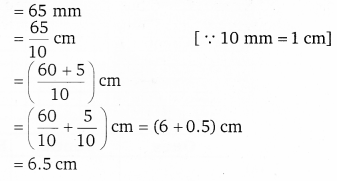# Class 6 Maths NCERT Solutions for Chapter 8 Decimals Ex – 8.1

## Decimals

Question 1.
Write the following as numbers in the given table:Solution:
On filling the given table, we haveQuestion 2.
Write the following decimals in the place value table:

(a) 19.4
(b) 0.3
(c) 10.6
(d) 205

Solution:
Let us make a common place value table, assigning appropriate place value to the digits in the given numbers. We have,Question 3.
Write each of the following as decimals:

(a) Seven- tenths
(b) Two tens and nine-tenths
(c) Fourteen point six
(d) One hundred and two ones
(e) Six hti red point eight

Solution:
(a) Seven-tenths =$\frac { 7 }{ 10 }$ = 0.7
(b) Two tens, 9 – tenths = 20 +$\frac { 9 }{ 10 }$ = 20.9
(c) Fourteen point six = 14.6
(d) One Hundred and 2-ones = 1 x 100 + 0 x 10 + 2 x 1 + 0 x$\frac { 1 }{ 10 }$
= 100 + 0 + 2 = 102.0
(e) Six hundred point eight = 600.8

Question 4.
Write each of the following as decimals: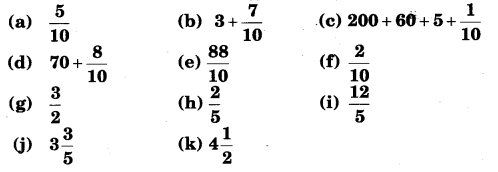Solution: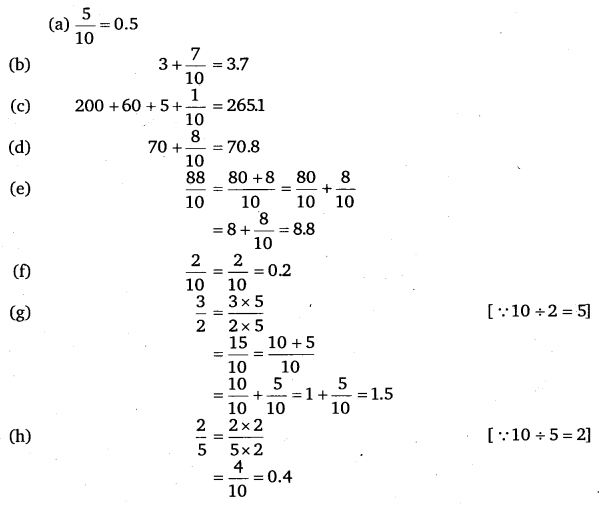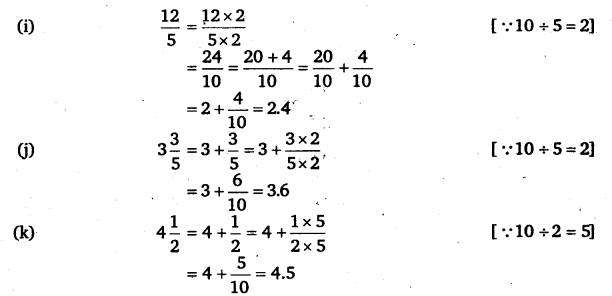Question 5.
Write the following decimals as fractions. Reduce the fractions to lowest form:

(a) 0.6
(b) 2.5
(c) 1.0
(d) 3.8
(e) 13.7
(f) 21.2
(g) 6.4

Solution:Question 6.
Express the following as cm vising decimals:

(a) 2 mm
(b) 30 mm
(c) 116 mm
(d) 4 cm 2mm
(e) 162 mm
(f) 83 mm

Solution: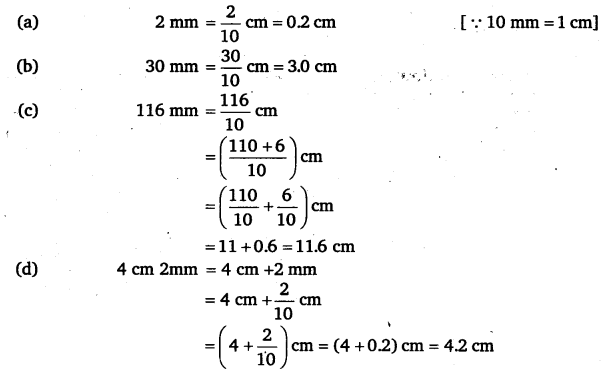Question 7.
Between which two whole numbers on the number line are the given numbers lie?

Which of these whole numbers is nearer the number?(a) 0.8
(b) 5.1
(c) 2.6
(d) 6.4
(e) 9.1
(f) 4.9

Solution:Question 8.
Show the following numbers on the number line.

(a) 0.2
(b) 1.9
(c) 1.1
(d) 2.5

Solution:
(a)
Since 0.2 > 0 but <1. There are 2 tenth in it. Divide the unit length between 0 and 1 into 10 equal parts and take 2 parts as shown below. Thus, A represents 0.2.

(b)
Since 1 < 1.9 < 2. Divide the unit length between 1 and 2 into 10 equal parts and take 9 parts as shown below. Thus, A represents 1.9.

(c)
Since 1 < 1.1 < 2. Divide the unit length between 1 and 2 into 10 equal parts and take 1 part as shown below. Thus, A represents 1.1.

(d)
Since 2 < 2.5 < 3. Divide the unit length between 2 and 3 into 10 equal parts and take 5 parts as shown below. Thus, A represents 2.5.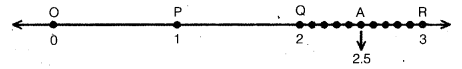Question 9.
Write the decimal number represented by the points A, B, C, D on the given number lineSolution:
Since A lies between 0 and 1 and the unit length between 0 and 1 stands divided in 10 equal parts. Also A is at the 8th point.
Thus, A represents 0.8.
Since, B lies between 1 and 2 and the unit length between 1 and 2 stands divided in 10 equal parts. Also, B lies 3 points ahead of 1. Therefore, B represents 1.3.
Since C and D lies between 2 and 3 and the unit length between 2 and 3 stands divided between 2 and 3 in 10 equal parts. Also C and D respectively lies 2 and 9 points ahead of 2.
∴ C represents 2.2 and D represents 2.9.

Question 10.
(a)
The length of Ramesh’s notebook is 9 cm 5 mm. What will be its length in cm?
(b) The length of a young gram plant is 65 mm. Express its length in cm.

Solution:
(a)
Length of Ramesh’s notebook(b) Length of gram plant